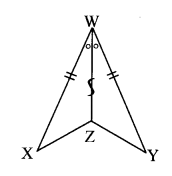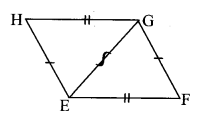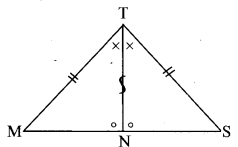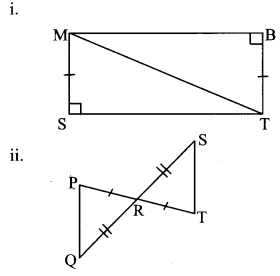SSC BOARD PAPERS IMPORTANT TOPICS COVERED FOR BOARD EXAM 2024

### chapter 13 - Congruence of triangles Balbharati solutions for Mathematics 8th Standard Maharashtra State Board

PRACTICE SET 13.1 [PAGE 85]

### Balbharati solutions for Mathematics 8th Standard Maharashtra State Board Chapter 13 Congruence of triangles Practice Set 13.1 [Page 85]

Practice Set 13.1 | Q 1.1 | Page 85

In each pair of triangles in the following figures, parts bearing identical marks are congruent. State the test and correspondence of vertices by which triangles in each pair are congruent.

Solution:
i.The two triangles are congruent by SAS test in the correspondence XWZ ↔ YWZ.

ii.The two triangles are congruent by hypotenuse-side test in the correspondence KJI ↔ LJI.

iii.The two triangles are congruent by SSS test in the correspondence HEG ↔ FGE.

iv.

The two triangles are congruent by ASA test is the correspondence SMA ↔ OPT.

v.The two triangles are congruent by ASA test or SAS test or SAA test in the correspondence MTN ↔ STN.

PRACTICE SET 13.2 [PAGE 87]

### Balbharati solutions for Mathematics 8th Standard Maharashtra State Board Chapter 13 Congruence of triangles Practice Set 13.2 [Page 87]

Practice Set 13.2 | Q 1.1 | Page 87

In the  triangle given below, parts shown by identical marks are congruent. State the test and the one to one correspondence of vertices by which triangles in each pair are congruent and remaining congruent parts.Solution:
i. In ∆MST and ∆TBM,
∴ side MS ≅ side TB … [Given]
m∠MST = m∠TBM = 90° … [Given]
hypotenuse MT ≅ hypotenuse MT
…[Common side]
∴ ∆MST ≅ ∆TBM …[by hypotenuse-side test]
∴ side ST ≅ side BM …[Corresponding sides of congruent triangles]
∠SMT ≅ ∠BTM …[Corresponding sides of congruent triangles]
∠STM ≅ ∠BMT …[Corresponding sides of congruent triangles]

ii. In ∆PRQ and ∆TRS,
side PR ≅ side TR … [Given]
∠PRQ ≅ ∠TRS …[Vertically opposite angles]
side RQ ≅ side RS … [Given]
∴ ∆PRQ ≅ ∆TRS …[by SAS test]
∴ side PQ ≅ side TS …[Corresponding sides of congruent triangles]
∠RPQ ≅ ∠RTS …[Corresponding sides of congruent triangles]
∠PQR ≅ ∠TSR …[Corresponding sides of congruent triangles]

iii. In ∆DCH and ∆DCF,
∠DCH ≅ ∠DCF …[Given]
∠DHC ≅ ∠DFC …[Given]
side DC ≅ side DC …[Common side]
∴ ∆DCH ≅ ∆DCF …[by AAS test]
∴ side HC ≅ side FC …[Corresponding sides of congruent triangles]
side DH ≅ side DF…[Corresponding sides of congruent triangles]
∠HDC ≅ ∠FDC ….[Corresponding sides of congruent triangles]

Practice Set 13.2 | Q 2.1 | Page 87

In the adjacent figure, seg AD ≌ seg EC Which additional information is needed to show that ∆ ABD and ∆ EBC will be congruent by A-A-S test ?Solution:
In ∆ABD and ∆CBE,
∴ seg AD ≅ seg CE …[Given]
∠ABD ≅ ∠CBE …[Vertically opposite angles]
∴ The necessary condition for the two triangles to be congruent by AAS test is